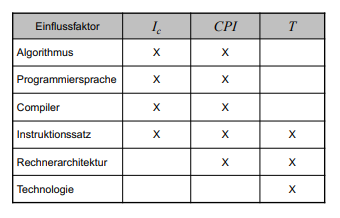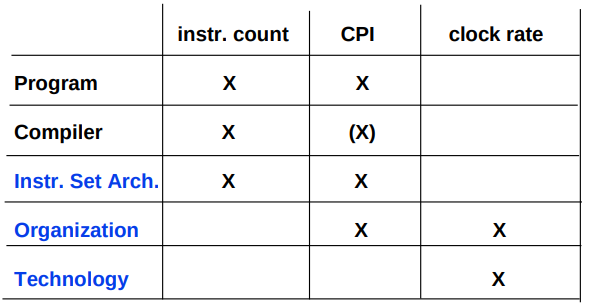#> RESTKHZ _

# 计算机性能评估

休止千鹤  |    27/02/2022

## 性能 Performance

• 执行时间 Execution time $[s]$: 一个给定的程序需要多长时间执行完
• 吞吐量 Throughput $[s^{-1}]$: 在单位时间内可以处理多少任务

$Performance_x \sim \frac{1}{ExTime_x}$

$\frac{ExTime_A}{ExTime_B}=\frac{Performance_B}{Performance_A}$

## 时间测量

### Elapsed time

Elapsed time: elapsed real time (or simply real time, or wall-clock time) is the time taken from the start of a computer program until the end as measured by an ordinary clock. Elapsed real time includes I/O time, any multitasking delays, and all other types of waits incurred by the program. (Wikipedia)

### CPU time [Prozessorausführungszeit]

• user CPU time 在程序上花费的时间(用户空间)
• system CPU time 这个程序在系统调用花费的时间(内核空间)

CPU time = usr+sys

## 执行时间计算

$T_{exe}=I_c \times CPI \times T$

• $T_{exe}$: 一个程序的 CPU time
• $I_c$: 执行时处理的机器指令数量
• $CPI$: Cycle per instruction 平均处理每个指令需要多少周期
• $T$: 处理器时钟频率, 主频clock rate和Instr. Set Arch. 可能有关系, 不知道这个课件是否出现错误.### 关于CPI

$CPI=\sum^{n}_{i=1}(CPI_i\cdot F_i)$

Store占10%,CPI=2

CPI=1.5

## MIPS-Rate (million instructions per second)

MIPS-Rate常用作产品指标.(某大学课件: (很可惜)被经常作为常用指标)

$MIPS-Rate \sim \frac{I_c}{ T_{exe} \times 10^6 }$

Peak-MIPS 则选择CPI最小的一组指令, 实际应用参考价值不高. 就像考试出的题都是找你会的出的. 这样的考试除了图一乐没有意义.

MFLOPS通常是高性能机器的指标.

## Benchmarks

Benchmarks之于机器也有缺陷, 就像凯氏定氮法之于奶粉一样…

## Amdahls定律

[=========|==part F==|============]

$S=\frac{F}{F_{opt}}$

$SpeedUp=\frac{1}{(1-F)+\frac{F}{S}}$

Views:Categories

ai:

"\"这个符号应该是除号吧？